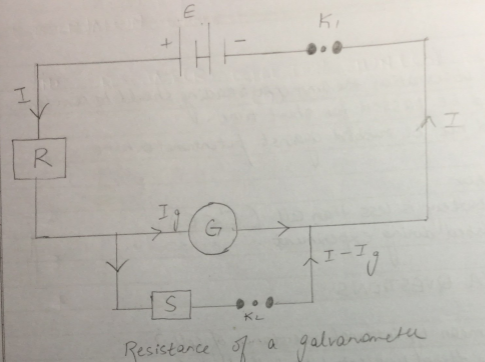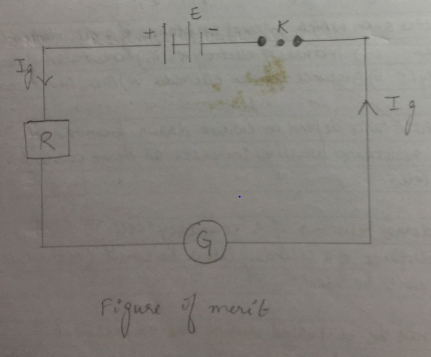# Determining resistance of a galvanometer by half deflection method and to find its figure of merit .

Aim : To determine resistance of a galvanometer by half deflection method and to find its figure of merit .

Apparatus : A Weston type of galvanometer , a voltmeter , a battery /battery eliminator , two 10,000 ohm and 200 ohm ) resistance boxes , two one way key , a rheostat , a screw gauge , a meter scale , an ammeter of given range , connecting wires and a piece of sand paper .

Theory

The resistance of the given galvanometer as found by half deflection method .

G= (RxS)/(R-S) (1)

Where R is the resistance connected in series with the galvanometer and S is the shunt resistance .

The figure of merit , K =E /[(R+G)Q] (2)

Where E is the end of the cell and Q is the deflection produced with resistance R.

The maximum current that can pass through the galvanometer , Ig=nk

Where n is the total number of dimension on the galvanometer scale on either side of zero .

Procedure

1. Resistance of galvanometer by half deflection method

• Make the connections accordingly as shown in circuit diagram .
• See that all plugs of the resistance boxes are tight
• Take out the high resistance (200 Ohm ) from the resistance box R and insert key K1 only .
• Adjust the value of R1 so that deflection is maximum , even in number and within the scale .
• Note the deflection . Let it be Q.
• Insert the key k2 also and without changing the value of R2 ,adjust the value of S , such that deflection in the galvanometer reduces to exactly half the value of resistance S.
• Note the value of resistance S .
• Repeat step 4 & 7 three times taking different R and adjusting .

2. Figure of merit

• Take one cell of the battery and find its emf by a voltmeter by connecting +ve of the voltmeter with +ve of cell and – ve of the voltmeter with -ve of cell . Let it be E.
• Take connection as in circuit diagram .
• Adjust the value of R to obtain a certain deflection Q when circuit is closed .
• Note the value of resistance R and deflection Q.
• Now change the value of R and note the galvanometer deflection again .
• Repeat the step with both cells of the battery with different voltages like 2,4,6 ,8 volts from battery eliminator.
• Find the figure of merit K using the formula

Observation table

 Resistance (R) Deflection (Q) Shunt (S) G =(RxS)/(R-S) 5000 10 5 5.005 10000 6 3 3 2000 20 10 10.05 1500 26 3 13.11 Average G = 7.79 ohms
 Resistance (R) Deflection (Q) K 5000 9 0.556 10000 5 0.60 2000 20 0.502 1500 25 0.524 Average K = 0.5455 A/dn

Calculations

1. G = (5.005+3+10.05+13.11)/4 [E = 1.45V]
2. Figure of Merit : Current in the galvanometer per unit time
3. K = IQ = [(E)/(R+G)]x[1/Q] – SI unit = AD division = 10-5 Al div

Result

Resistance of given galvanometer is 7.79 ohm .

Figure of merit of given galvanometer is 0.545 A/dn .

Precautions

All connections should be neat and tight .

The emf of cell / battery should be constant .

Source of error

The scene of the instrument may be loose .

The galvanometer division may not be of equal size.

Diagram

Resistance of GalvanometerFigure of MeritViva question

What is the galvanometer ?

It is a device used for detecting feeble electric currents in circuit .

Define the figure of merit of a galvanometer ?

The quantity of current required to produce a deflection of one division in the galvanometer is called figure of merit of a galvanometer . It is represented by K . Its unit is ampere per division .

Why is this method called half deflection method ?

It is so because the deflection is made half by using a shunt resistance S.

Under what condition id the resistance of a galvanometer (E1) equal to shunt resistance (S)?

E1=S , only when series resistance R is very high .

You can also get Class XII Practicals on BiologyPhysics, and Physical Education.

## 21 Replies to “Determining resistance of a galvanometer by half deflection method and to find its figure of merit .”

1.Dhanishta says:

Need to see graph

1.iamdivyanshushekhar@gmail.com says:

Thank you

2.iamdivyanshushekhar@gmail.com says:

Soapstone first-aid

2.Ayaan Sayyed says:

Need to update I think it’s older version some coloum are missing in observations table

3.Rajput says:

Thnku so much..ur an angel..really love u

4.chandani says:

thank you soooo much ,

5.sourabh haldar says:

Thsnks for suggestion

6.Vishvendra says:

You have not told emf of the cell

7.Ankita Sahu says:

Thank you… It is quite helpful.

8.Rounik says:

Wo
Awesome work

9.Bhabasagar Bag says:

Experiment=5

10.Bhosadiwala says:

Thanks

11.Akshar Patel says:

Thanks

1.Golam Rabbi says:

I need a discussions …please give me a discussions for this Experiment

12.Golam Rabbi says:

I need a discussions …please give me a discussions for this Experiment

13.Praveen Raj says:

Plz check the observation table and update because some column are missing 🙁

14.SAKSHI PATHIK says:

Very much thankful to have this…

15.Karan says:

Nice works

16.Karan says:

Nice works

17.Hiral patel says:

Awesome work
Really thankful to have it

18.Hiral patel says:

Fantastic work
Nice to have it